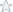# Straigherline MAT 251 Calulus 2 Unit 6 Test Solutions

Please see attached file for formatted questions

===============================================================
Review Test Submission: Graded Exam - Unit 6
Test Graded Exam - Unit 6
Status Completed
Score 90 out of 150 points

Question 2
A tank is formed by revolving the graph of 4 around they-axis. The tank has a height of 16. Find
y=x
the value of m such that the line y = m represents the height at which the tank is half full. Round your

Question 3
Given the differential equation = f(x, y) and Initial condition y(xo) =-yo. Euler's
method uses the following equation to approximate successive points on the solution
curve:

Question 4
Particles A and B are located on the x axis • The masses and positions of the partldes
are given by:

What is the center of mass ot this two particle system?

Question 5
An object is moving along the coordinate line with velocity v(t) = 5 cost, 0 \$ 1\$ 21t.
What is the object's displacement during the interval from t= 0 to/= 21t?

Question 6
If an object is displaced a distanced by a constant force F, then the amount ofworl< done
is given by the equation:

Question 7
Which of the following integrals represents the area of the surface fanned by revolving the

Question 9
Suppose that a force of 20 N stretches a spring 0.4 m beyond its natural length.

Question 10
Find the volume of the solid obtained by rotating the area bounded by y = sinx and the
x -axis on the interval 0 sx s 21t about the x-axis .

Question 11
Given the differential equation ~ =x + y and Initial point ( 0, 0 ), use Euler's method with
step size l!.x = 0.5 to approximate the coordinates of the next three points.
(x3,y3)=

Question 12
Suppose that f(x) is the probability density function describing the distribution for the
number of minutes H tekes to solve a puzzle.
Which of the following represents the probability that you witl solve the puzzle in 8·10
minutes?

Question 13
Find the length of the arc:
x=3cost+ I, y =3sint-S, OstS!t

Question 15
A projectile Is shot Into the air with lnital speed vo = 40 and angle of elevation 9=~What
is the position of the projectile at t = 3 ?

Question 16
Which of the following differential equations is NOT separable?

Question 17 0 out of 7.5 points
A solid has a flat base. which is bounded by the graph of y = :; and y = .Jx in the first quadrant only.
Each cross section of the solid p erpen dicula r to the x- axis is the shape of a square. Find the value of k

Question 18 0 out of 7.5 points
Given a curve defined by the parametric equations:
y =3t
The area under the curve from t = 0 to t = 3 is given by:

Question 19
Suppose we have a rod that is 6 meters long, with density function p(x) = 2x + 3.
What Is the center of mass of the rod?

Solutions are NOT perfect see file for score report 90/150

Subjects: Calculus II, Intermediate Calculus
Tags:

### Straighterline, Blackboard MAT251

Price\$9.95

Math Genius
 Shirley B. Member Since: Nov 1998 Customer Rating: 97.8% Projects Completed: 2378 Total Earnings: *Private* +1 Ratings from clients: 578
Project Details
Subjects: Calculus II, Intermediate Calculus
Tags:

## Straighterline, Blackboard MAT251

Customer Reviews
Rated 1 Times
Rating( 3 / 5 Stars)

Reviews of the solutions left by other users
No customer has left a detailed review for these math solutions. Be the first one to purchase them and leave a review for others!

Not exactly what you are looking for?
We regularly update our math homework solutions library and are continually in the process of adding more samples and complete homework solution sets. If you do not find what you are looking for, just go ahead and place an order for a custom created homework solution. You can hire/pay a math genius to do your homework for you exactly to your specifications.